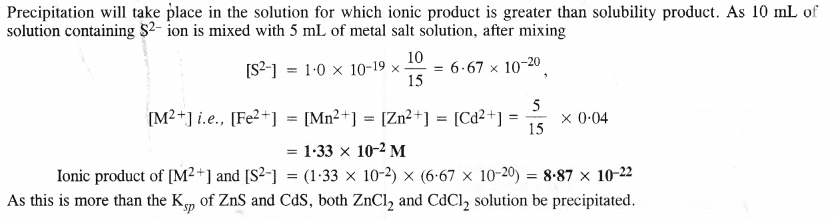## NCERT Solutions for Class 11 Chemistry Chapter 7 Equilibrium

These Solutions are part of NCERT Solutions for Class 11 Chemistry. Here we have given NCERT Solutions for Class 11 Chemistry Chapter 7 Equilibrium.

Question 1.
A liquid is in equilibrium with its vapours in a sealed container at a fixed temperature. The volume of the container is suddenly increased,
(i) What is the initial effect of the change on the vapour pressure ?
(ii) How do the rates of evaporation and condensation change initially ?
(iii) What happens when equilibrium is restored finally and what will be the final vapour pressure ?
(i) On increasing the volume of the container, the vapour pressure will initially decrease because the same amount of vapours are now distributed over a larger space.
(ii) On increasing the volume of the container, the rate of evaporation will increase initially because now more space is available. Since the amount of the vapours per unit volume decrease on increasing the volume, therefore, the rate of condensation will decrease initially.
(iii) Finally, equilibrium will be restored when the rates of the forward and backward processes become equal. However, the vapour pressure will remain unchanged because it depends upon the temperature and not upon the volume of the container.

Question 2.
What is Kc for the following reaction in a state of equilibrium :
2SO2(g) + O2(g) $$\rightleftharpoons$$ 2SO3(g) ?
Given : [SO2] = 0.6 M ; [O2]= 0.82 M ; and [SO3] = 1.90 M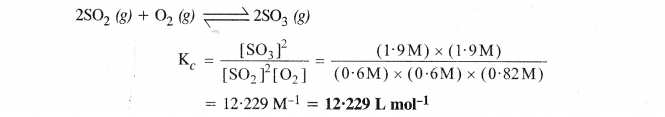Question 3.
At certain temperature and total pressure of 105Pa, iodine vapours contain 40t% by volume of iodine atoms in the equilibrium I2 (g) $$\rightleftharpoons$$ 2I (g). Calculate Kp for the equilibrium.
According to available data :
Total pressure of equilibrium mixture = 105 Pa
Partial pressure of iodine atoms (I) = $$\frac { 40 }{ 100 }$$ × (105 Pa) = 0.4 × 105 Pa
Partial pressure iodine molecules (I2) = $$\frac { 60 }{ 100 }$$ × (105 Pa) = 0.6 × 105 Pa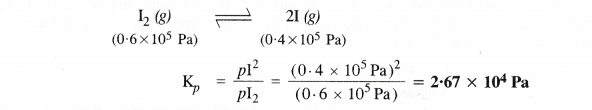Question 4.
Write the expression for the equilibrium constant for the following reactions
(i) 2NOCl(g)) $$\rightleftharpoons$$ 2NO(g) + Cl2(g)
(ii) 2CU(N03)2(s) $$\rightleftharpoons$$ 2CuO(s) + 4NO2(g) + O2(g)
(iii) CH3COOC2H5(aq) + H2O(l) $$\rightleftharpoons$$ CH3COOH(aq) + C2H5OH(aq)
(iv) Fe3+(aq) + 3OH(aq) $$\rightleftharpoons$$ Fe(OH)3(s)
(v) I2(s) + 5F2(g) $$\rightleftharpoons$$ 2IF5(l).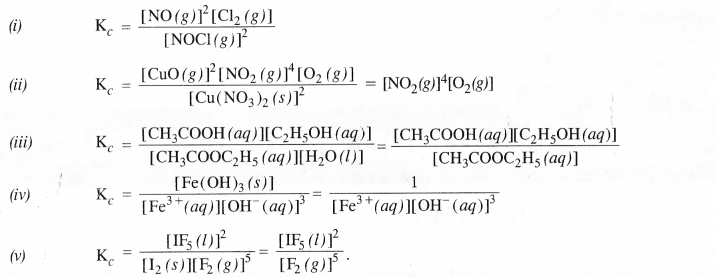Question 5.
Find the value of Kc for each of the following equilibria from the value of Kp:
(a) 2NOCl (g) $$\rightleftharpoons$$ 2NO (g) + Cl2 (g) ; Kp = 1.8 x 10-2 atm at 500 K
(b) CaCO3 (s) $$\rightleftharpoons$$ CaO (s) + CO2 (g) ; Kp = 167 atm at 1073 K.
Kp and Kc are related to each other as Kp = Kc ( RT)∆ng
The value of Kc can be calculated as follows :
(a) 2NOCl (g) $$\rightleftharpoons$$ 2NO (g) + Cl2 (g)
Kp = 1.8 × 10-2 atm,
ng = 3 – 2 = 1 ; R = 0.0821 litre atm K-1 mol-1 ; T = 500 K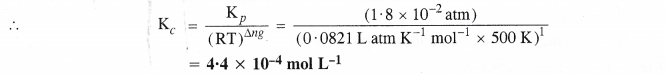(b) CaCO3 (s) $$\rightleftharpoons$$ CaO (s) + CO2 (g)
Kp = 167 atm, ∆ng = 1 – 0 = 1
R = 0.0821 liter atm K-1 mol-1 ; T = 1073 KQuestion 6.
For the following equilibrium, Kc = 6.3 × 1014 at 1000 K
NO (g) + O3 (g) $$\rightleftharpoons$$ NO2(g) + O2 (g)
Both the forward and reverse reactions in the equilibrium are elementary bimolecular reactions. What is Kc for the reverse reaction ?
For the reverse reaction Kc = $$\frac { 1 }{ { K }_{ c } }$$ = $$\frac { 1 }{ 6.3\times { 10 }^{ 14 } }$$ = 1.59 × 10-15

Question 7.
Explain why pure liquids and solids can be ignored while writing the value of the equilibrium constant.
In writing the expression for the equilibrium constant, molar concentrations of the species involved in the reaction are taken into account.

We all know that the molar concentration of a substance implies number of moles per unit volume (moles/volume) or (mass/volume).

Since mass divided by volume represents density of the substance therefore, molar concentration of a substance is directly related to its density.
Molar concentration = $$\frac { No.ofmoles }{ Volume }$$ α $$\frac { Mass }{ Volume }$$ (α density)
Density as we know, is an intensive property and does not depend upon the mass of the substance.

Consequently, molar concentration of a pure substance (solid or liquid) has always the same value and it can be ignored while writing the value of the equilibrium constant.

However, for substances in gaseous state or in aqueous solution, the amount in a given volume can vary and their molar concentration does not remain constant and cannot be ignored while writing the expression for the equilibrium constant.

Question 8.
Reaction between nitrogen and oxygen takes place as follows :
2N2 (g) + O2 (g) $$\rightleftharpoons$$ 2N2O (g)
If a mixture of 0.482 mol of N2 and 0.933 mol of O2 is placed in a reaction vessel of volume 10 L and allowed to form N20 at a temperature for which Kc = 2.0 × 10-37, determine the composition of the equilibrium mixture.
Let x moles of N2 (g) take part in the reaction. According to the equation, $$\frac { x }{ 2 }$$ moles of O2 (g) will react to form x moles of N2O (g). The molar concentration per litre of different species before the reaction and at the equilibrium point is :The value of equilibrium constant (2.0 × 10-37) is extremely small. This means that only small amounts of reactants have reacted. Therefore, x is extremely small and can be omitted as far as the reactants are concerned.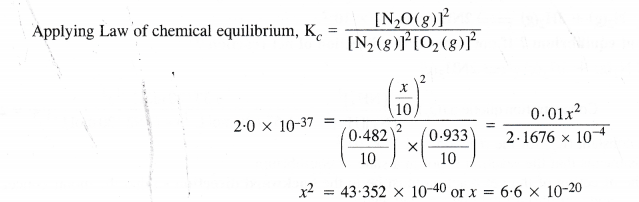As x is extremely small, it can be neglected.
Thus, in the equilibrium mixture
Molar cone. of N2 = 0.0482 mol L-1
Molar cone. of O2 = 0.0933 mol L-1
Molar cone. of N2O = 0.1 × x = 0.1 × 6.6 10-20 mol L-1
= 6.6 × 10-21

Question 9.
Nitric oxide reacts with bromine and gives nitrosyl bromide as per reaction given below :
2NO (g) + Br2 (g) $$\rightleftharpoons$$ 2NOBr (g)
When 0.087 mole of NO and 0.0437 mole of Br2 are mixed in a closed container at constant temperature, 0.0518 mole of NOBr is obtained at equilibrium. Determine the composition of the equilibrium mixture.
The balanced chemical equation for the reaction is :
2NO (g) + Br2 (g) $$\rightleftharpoons$$ 2NOBr (g)
According to the equation, 2 moles of NO (g) react with 1 mole of Br2 (g) to form 2 moles of NOBr (g). The composition of the equilibrium mixture can be calculated as follows :
No. of moles of NOBr (g) formed at equilibrium = 0.0518 mol (given)
No. of moles of NO (g) taking part in reaction = 0.0518 mol
No. of moles of NO (g) left at equilibrium = 0.087 – 0.0518 = 0.0352 mol
No. of moles of Br2 (g) taking part in reaction = $$\frac { 1 }{ 2 }$$ × 0.0518 = 0.0259 mol
No. moles of Br2 (g) left at equilibrium = 0.0437 – 0.0259 = 0.0178 mol
The initial molar concentration and equilibrium molar concentration of different species may be represented as :Question 10.
At 450 K, Kp = 2.0 × 1010 bar-1 for the equilibrium reaction :
2SO2 (g) = O2 (g) $$\rightleftharpoons$$ 2SO3(g)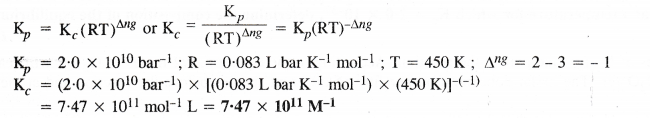Question 11.
A sample of HI (g) is placed in a flask at a pressure of 0.2 atm. At equilibrium partial pressure of HI (g) is 0.04 atm. What is Kp for the given equilibrium ?
2HI (g) $$\rightleftharpoons$$ H2(g) + I2(g)
pHI = 0.04 atm, pH2 = 0.08 atm ; pI2 = 0.08 atm
Kp = $$\frac { p{ H }_{ 2 }\times p{ I }_{ 2 } }{ { p }_{ HI }^{ 2 } }$$ = $$\frac { (0.08atm)\times (0.08atm) }{ 0.04atm)\times (0.04atm) }$$ = 4.0

Question 12.
A mixture of 1.57 mol of N2, 1.92 mol of H2 and 8.13 mol of NH3 is introduced into a 20 L reaction vessel at 500 K. At this temperature, the equilibrium constant Kc for the reaction given as follows :
N2(g) + 3H2(g) $$\rightleftharpoons$$ 2NH3(g) is 1.7 × 10-2.
Is this reaction at equilibrium ? If not, what is the direction of net reaction ?
The reaction is : N2(g) + 3H2(g) $$\rightleftharpoons$$ 2NH3(g)

The equilibrium constant (Kc) for the reaction = 1.7 X 10-2
As Qc, ≠ Kc ; this means that the reaction is not in a state of equilibrium.
As Qc > Kc ; the direction of the net reaction must be in the backward direction so that the molar concentration of NH3 may decrease and equilibrium may be attained again.

Question 13.
The equilibrium constant expression for a gas reaction is,Write the balanced chemical equation corresponding to this expression.
Balanced chemical equation for the reaction is
4 NO(g) + 6 H2O (g) $$\rightleftharpoons$$ 4NH3 (g) + 5 O2(g)

Question 14.
If 1 mole of H2O and 1 mole of CO are taken in a 10 litre vessel and heated to 725 K, at equilibrium point 40 percent of water (by mass) reacts with carbon monoxide according to equation!
H2O(g) + CO(g) $$\rightleftharpoons$$ H2(g) + CO2(g)
Calculate the equilibrium constant for the reaction.
Number of moles of water originally present = 1 mol
Percentage of water reacted =40%
Number of moles of water reacted = $$\frac { 1\times 40 }{ 100 }$$ = 0.4 mol
Number of moles of water left = (1 – 0.4) = 0.6 mol
According to the equation, 0.4 mole of water will react with 0.4 mole of carbon monoxide to form 0.4 mole of hydrogen and 0.4 mole of carbon dioxide.
Thus, the molar cone./litre per litre of the reactants and products before the reaction and at the equilibrium point are as follows :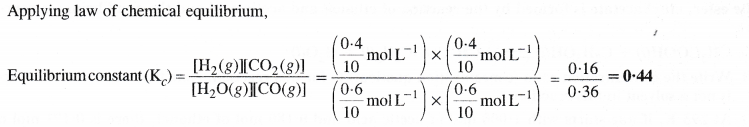Question 15.
At 700 K, the equilibrium constant for the reaction H2(g) + I2(g) $$\rightleftharpoons$$ 2HI(g) is 54.8. If 0.5 mol L-1 of HI (g) is present at equilibrium at 700 K, what are the concentration of H2(g) and I2(g) ? Assume that we initially started with HI(g) and allowed it to reach equilibrium at 700 K.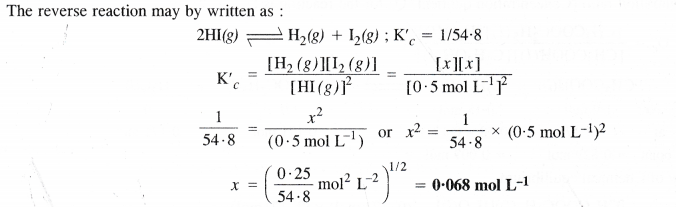Question 16.
What is the equilibrium concentration of each of the substances in the equilibrium when the initial concentration of ICl was 0.78 M ?
2ICl(g) $$\rightleftharpoons$$ I2(g) + Cl2(g) ; Kc = 0.14
Suppose at equilibrium, the molar concentration of both I2(g) and Cl2(g) is x mol L-1Question 17.
Kp = 0.04 atm at 898 K for the equilibrium shown below. What is the equilibrium concentration of C2H6 when it is placed in a flask at 4 atm pressure and allowed to come to equilibrium.
C2H6(g) $$\rightleftharpoons$$ C2H4(g) + H2(g)Question 18.
The ester, ethyl acetate is formed by the reaction of ethanol and acetic acid and the equilibrium is represented as :
CH3COOH(l) + C2H5OH(l) $$\rightleftharpoons$$ CH3COOC2H5(l) + H2O(l)
(i) Write the concentration ratio (concentration quotient) Q for this reaction. Note that water is not in excess and is not a solvent in this reaction.
(ii) At 293 K, if one starts with 1.000 mol of acetic acid and 0.180 mol of ethanol, there is 0.171 mol of ethyl acetate in the final equilibrium mixture. Calculate the equilibrium constant.
(iii) Starting with 0.50 mol of ethanol and 1.000 mol of acetic acid and maintaining it at 293 K, 0.214 mol of ethyl acetate
is found after some time. Has equilibrium been reached ?Question 19.
A sample of pure PCl5 was introduced into an evacuated vessel at 473 K. After equilibrium was reached, the concentration of PCl5 was found to be 0.5 x 10-1 mol L-1. If Kc is 8.3 × 10-3, what are the concentrations of PCl3 and Cl2 at the equilibrium ?
Let the initial molar concentration of PCl5 per litre = x mol
Molar concentration of PCl5 at equilibrium = 0.05 mol
∴ Moles of PCl55 decomposed = (x – 0.05) mol
Moles of PCl3 formed = (x – 0.05) mol
Moles of Cl2 formed = (x – 0.05) mol
The molar cone. /litre of reactants and products before the reaction and at the equilibrium point are: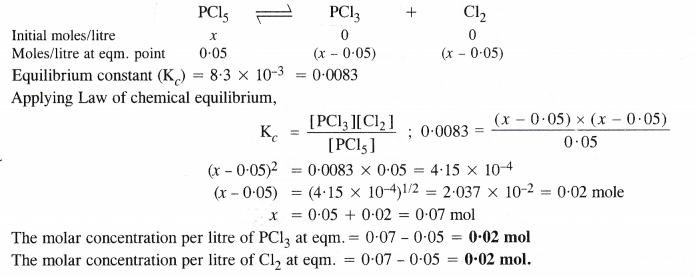Question 20.
One of the reactions that takes place in producing steel from iron ore is the reduction of iron (II) oxide by
carbon monoxide to give iron metal and CO2
FeO(s) + CO(g) $$\rightleftharpoons$$ Fe(s) + CO2(g); Kp = 0.265 atm at 1050 K
What are the equilibrium partial pressures of CO and CO2 at 1050 K if the initial pressures are :
Pco = 1.4 atm and Pco2 = 0.80 atm ?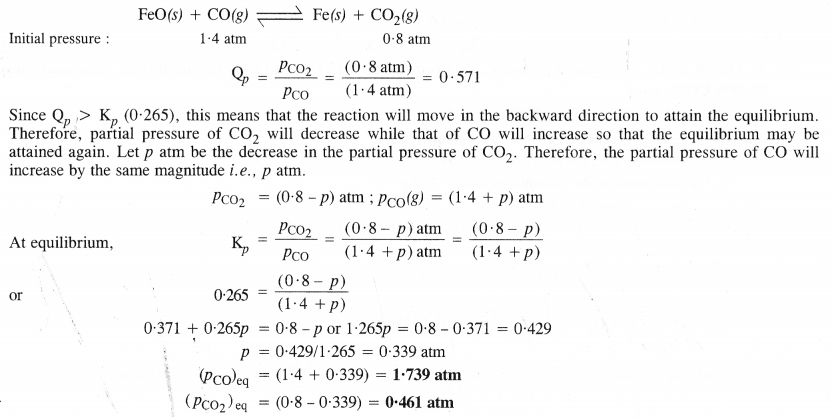Question 21.
Equilibrium constant Kc for the reaction, N2 (g) + 3H2 (g) $$\rightleftharpoons$$ 2NH3 (g) at 500 K is 0.061. At particular time, the analysis shows that the composition of the reaction mixture is : 3.0 mol L-1 of N2 ; 2.0 mol L-1 of H2 ; 0.50 mol L-1 of NH3. Is the reaction at equilibrium ? If not, in which direction does the reaction tend to proceed to reach the equilibrium ?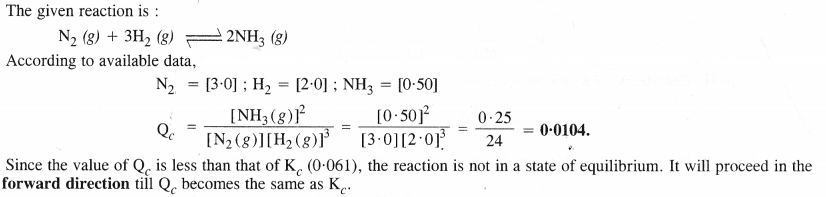Question 22.
Bromine monochloride (BrCl) decomposes into bromine and chlorine and reaches the equilibrium :
2BrCl (g) $$\rightleftharpoons$$ Br2 (g) + Cl2 (g)
The value of Kc is 32 at 500 K. If initially pure BrCl is present at a concentration of 3.3× 10-3 mol L-1, what is its molar concentration in the mixture at equilibrium ?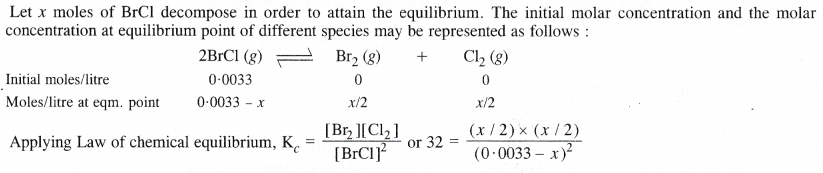Question 23.
At 1127 K and 1 atmosphere pressure, a gaseous mixture of CO and CO2 in equilibrium with solid carbon has 90.55% by mass.
C(s) + CO2(g) $$\rightleftharpoons$$ 2CO(g)
Calculate Kc for the reaction at the above temperature.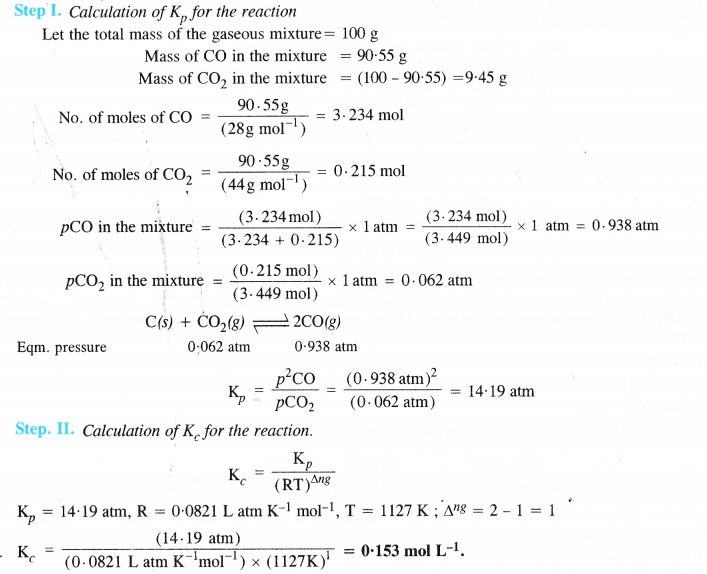Question 24.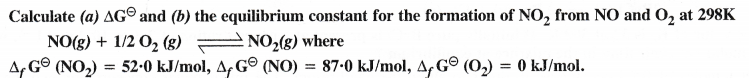Question 25.
Does the number of moles of reaction products increase, decrease or remain same when each of the following equilibria is subjected to a decrease in pressure by increasing the volume ?
(i) PCl5(g) $$\rightleftharpoons$$ PCl3(g) + Cl2
(ii) CaO(s) + CO2(g) $$\rightleftharpoons$$ CaCO3(s)
3Fe(s) + 4H2O(g) $$\rightleftharpoons$$ Fe3O4(s) + 4H2(g)
(i) Pressure will increase in the forward reaction and number of moles of products will increase.
(ii) Pressure will increase in backward reaction and number of moles of products will decrease.
(iii) The change in pressure will have no effect on the equilibrium constant and there will be no change in the number of moles.

Question 26.
Which of the following reactions will get affected by increase in pressure ? Also mention whether the change will cause the reaction to go to the right or left direction.Only those reactions will be affected by increasing the pressure in which the number of moles of the gaseous reactants and products are different (np -/ nr) (gaseous). With the exception of the reaction (1) ; all the remaining five reactions will get affected by increasing the pressure. In general,
• The reaction will gq to the left if np > nr.
• The reaction will go to the right if np < nr.
Keeping this in mind,
(i) Increase in pressure will not affect equilibrium because np = nr =3
(ii) Increase in pressure will favour backward reaction because np (2) > nr (1)
(iii) Increase in pressure will favour backward reaction because np (10) > nr (9)
(iv) Increase in pressure will favour forward reaction because np(1) < nr (2)
(v) Increase in pressure will favour backward reaction because np (2) > nr ( 1)
(vi) Increase in pressure will favour backward reaction because np (1) > nr (0).

Question 27.
The equilibrium constant for the following reaction is 1.6 x 105 at 1024 K.
H2(g) + Br2(g) $$\rightleftharpoons$$ 2HBr(g)
Find the equilibrium pressure of all gases if 10.0 bar of HBr is introduced into a sealed container at 1024 K.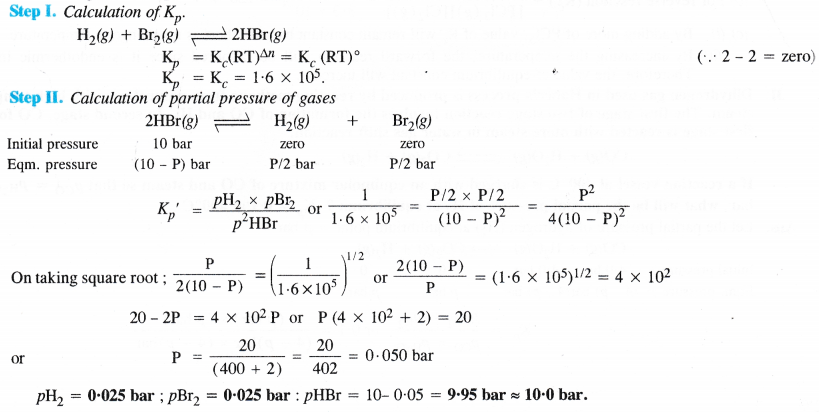Question 28.
Hydrogen gas is obtained from the natural gas by partial oxidation with steam as per following endothermic reaction :
CH4 (g) + H2O (g) $$\rightleftharpoons$$ CO (g) + 3H2 (g)
Write the expression for Kp for the above reaction
How will the value of Kp and composition of equilibrium mixture be affected by :
(i) increasing the pressure
(ii) increasing the temperature
(iii) using a catalyst ?
The expression for Kp for the reaction is :(i) By increasing the pressure, the no. of moles per unit volume will increase. In order to decrease the same, the equilibrium gets shifted to the left or in the backward direction. As a result, more of reactants will be formed and the value of Kp will decrease.
(ii) If the temperature is increased, according to Le Chatelier’s principle, the forward reaction will be favoured as it is endothermic. Therefore, the equilibrium gets shifted to the right and the value of Kp will increase.
(iii) The addition of catalyst will not change the equilibrium since it influences both the forward and the backward reactions to the same extent. But it will be attained more quickly.

Question 29.
What is the effect of :
(iii) removal of CO
(iv) removal of CH3OH
On the equilibrium 2H2 (g) + CO (g) $$\rightleftharpoons$$ CH3OH ?
(i) Equilibrium will be shifted in the forward direction.
(ii) Equilibrium will be shifted in the backward direction.
(iii) Equilibrium will be shifted in the backward direction.
(iv) Equilibrium will be shifted in the forward direction.

Question 30.
At 473 K, the equilibrium constant Kc for the decomposition of phosphorus pentachloride (PCl5) is 8.3 × 10-3. If decomposition proceeds as :
PCl5 (g) $$\rightleftharpoons$$ PCl3 (g) + Cl2 (g) ; ∆H = + 124.0 kJ mol-1
(a) Write an expression for Kc for the reaction.
(b) What is the value of Kc for the reverse reaction at the same temperature ?
(c) What would be the effect on Kc if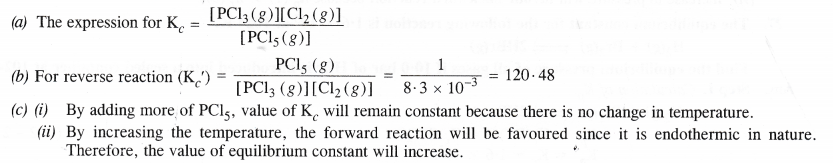Question 31.
Dihydrogen gas used in Haber’s process is produced by reacting methane from natural gas with high temperature steam. The first stage of two stage reaction involves the formation of CO and H2. In second stage, CO formed in first stage is reacted with more steam in water gas shift reaction.
CO(g) + H2O(g) $$\rightleftharpoons$$ CO2(g) + H2(g)
If a reaction vessel at 400°C is charged with an equimolar mixture of CO and steam so that pco = PH2O = 4.0 bar, what will be the partial pressure of H2 at equilibrium ? Kp = 0.1 at 400°C.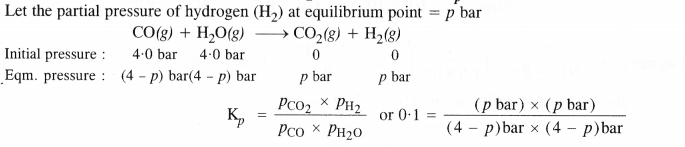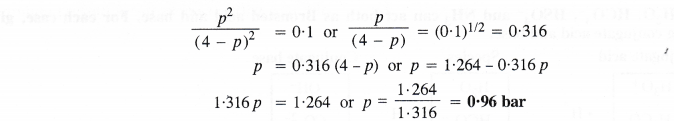Question 32.
Predict which of the following will have appreciable concentration of reactants and products :
(a) Cl2 (g) $$\rightleftharpoons$$ 2Cl (g) ; Kc = 5 ×10-39
(b) Cl2 (g) + 2NO (g) $$\rightleftharpoons$$ 2NOCl (g) ; Kc = 3.7 × 108
(c) Cl2 (g) + 2NO2 (g) $$\rightleftharpoons$$ 2NO2Cl (g) ; Kc = 1.8.
Following conclusions can be drawn from the values of Kc.
(a) Since the value of Kc is very small, this means that the molar concentration of the products is very small as compared to that of the reactants.
(b) Since the value of Kc is quite large, this means that the molar concentration of the products is very large as compared to that of the reactants.
(c) Since the value of Kc is 1.8, this means that both the products and reactants have appreciable concentration.

Question 33.
The value of Kc for the reaction 3O2(g) $$\rightleftharpoons$$ 2O3(g) is 2.0 × 1(10-50 at 25°C. If equilibrium concentration of O2 in air at 25°C is 1.6 × 10-2, what is the concentration of O2 ?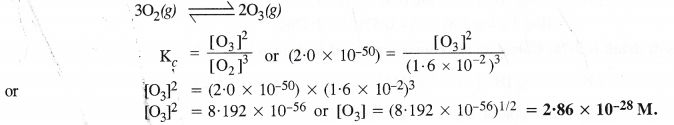Question 34.
The reaction CO(g) + 3H2(g) $$\rightleftharpoons$$ CH4(g) + H2O(g)
is at equilibrium at 1300 K in a 1L flask. It also contain 0.30 mol of CO, 0.10 mol of H2 and 0.02 mol of H2O and an unknown amount of CH4 in the flask. Determine the concentration of CH4 in the mixture. The equilibrium constant, Kc for the reaction at the given temperature is 3.90.Question .35.
What is meant by conjugate acid-base pair ? Find the conjugate acid/base for the following species :
HNO2,CN,HClO4,OH,CO32-,S2-.
An acid-base pair which differs by a proton only (HA $$\rightleftharpoons$$ N A + H+ ) is known as conjugate acid-base pair. Conjugate acid : HCN, H2O, HCO3, HS.
Conjugate base : NO2, ClO4, O2 .

Question 36.
Which of the following are Lewis Acids ?
H2O, BF3, H+ and NH4+
BF3, H+ , NH4+ ions are Lewis acids.

Question 37.
What will be the conjugate bases for the Bronsted acids ? HF, H2SO4 and H2CO3 ?
Conjugate bases : F, HSO4 , HCO3

Question 38.
Write the conjugate acids for the following Bronsted acids.
NH2, NH3 and HCOO
NH3, NH4+ and H2CO3.

Question 39.
The species H2O, HCO3, HSO4 and NH3 can act both as Bronsted acid and base. For each case, give the corresponding conjugate acid and base.Question 40.
Classify the following species into Lewis acids and Lewis bases and show how these can act as Lewis acid/Lewis base ?
(a) OH ions
(b) F
(c) H+
(d) BCl3
(a) OH ions can donate an electron pair and act as Lewis base.
(b) F ions can donate and electron pair and act as Lewis base.
(c) H+ ions can accept an electron pair and act as Lewis acid.
(d) BCl3 can accept an electron pair since Boron atom is electron deficient. It is a Lewis acid.

Question 41.
The concentration of hydrogen ions in a sample of soft drink is 3.8 × 10-3 M. What is its pH value ?
pH = – log [H+] = – log (3.8 × 10-3)
= (log 3 – log 3.8) = 3 – 0.5798 = 2.4202

Question 42.
The pH of soft drink is 3.76. Calculate the concentration of hydrogen ions in it.
pH = – log [H+] or log [H+] = -pH = – 3.76 = $$\bar { 4 }$$.24
[H+] = Antilog ($$\bar { 4 }$$.24) = 1.74 x 10-4M.

Question 43.
The ionisation constants of HF, HCOOH and HCN at 298 K are 6.8 x 10-4, 1-8 × 10-4 and 4.8 × 10-9 respectively. Calculate the ionisation constant of the corresponding congugate bases.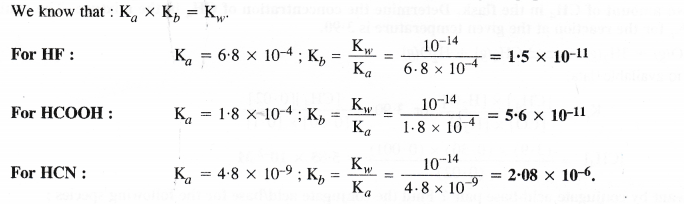Question 44.
The ionisation constant of phenol is 1.0 x 10-10. What is the concentration of phenate ion in 0.05 M solution of phenol and pH of solution ? What will be the degree of ionisation if the solution is also 0.01 M in sodium phenate ?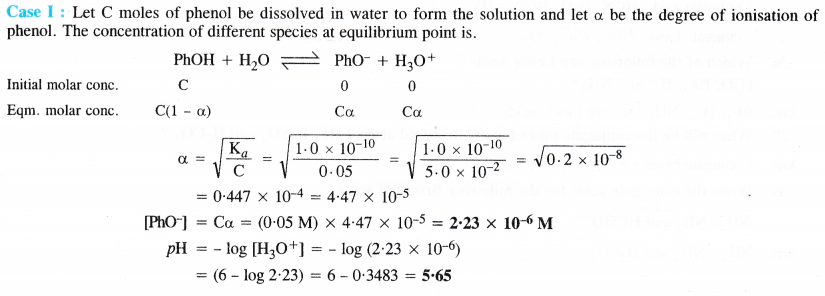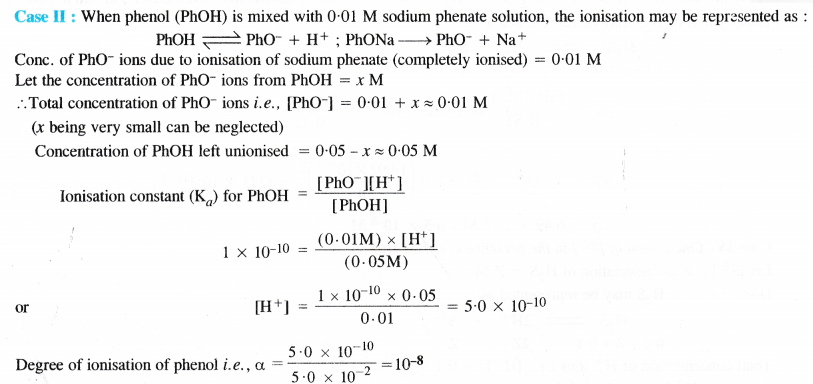Question 45.
The first dissociation constant H2S is 9.1 × 10-8. Calculate the concentration of HS ions in its 0.1 M solution and how much will this concentration be affected if the solution is 0.1 M in HCl also. If the second dissociation constant of H2S is 1.2 × 10-13, calculate the concentration of S2- ions under both the conditions.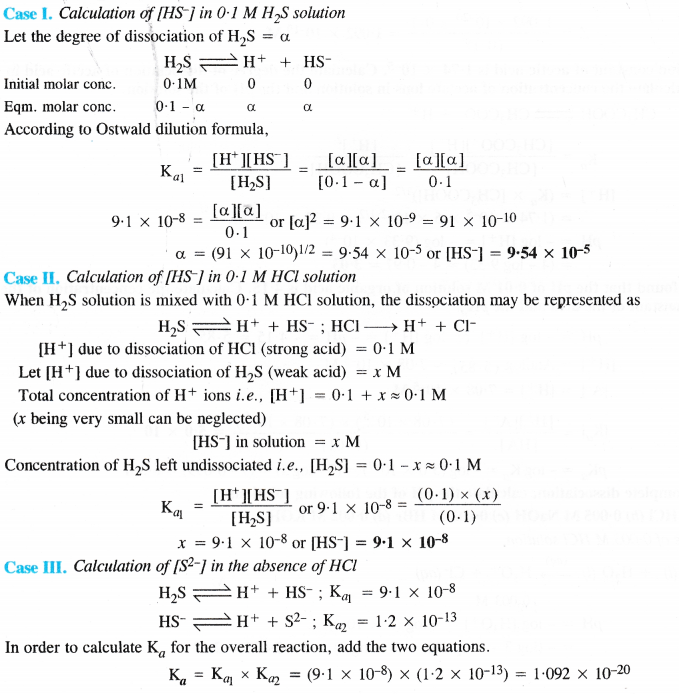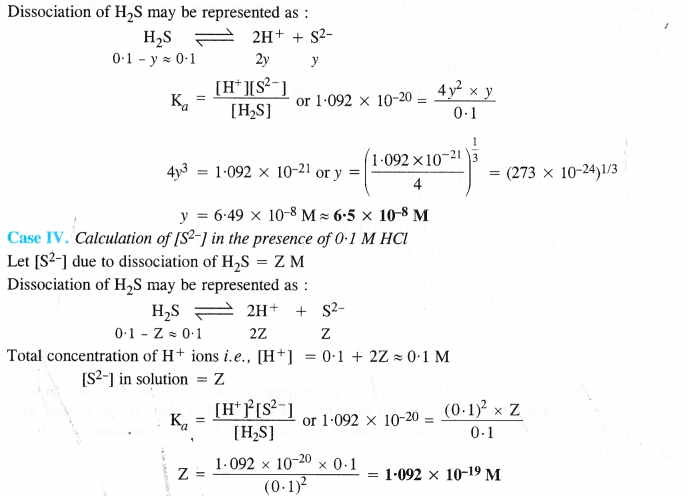Question 46.
The ionization constant of acetic acid is 1.74 × 10-5. Calculate the degree of dissociation of acetic acid in 0.05 M solution. Calculate the concentration of acetate ions in solution and the pH of the solution.Question 47.
It has been found that the pH of 0.01 M solution of organic acid is 4.15. Calculate the concentration of the anion, ionization constant of the acid and the pKa.Question 48.
Assuming complete dissociation, calculate the pH of the following solutions :
(a) 0.003 M HCl
(b) 0.005 M NaOH
(c) 0.002 M HBr
(d) 0.002 M KOH.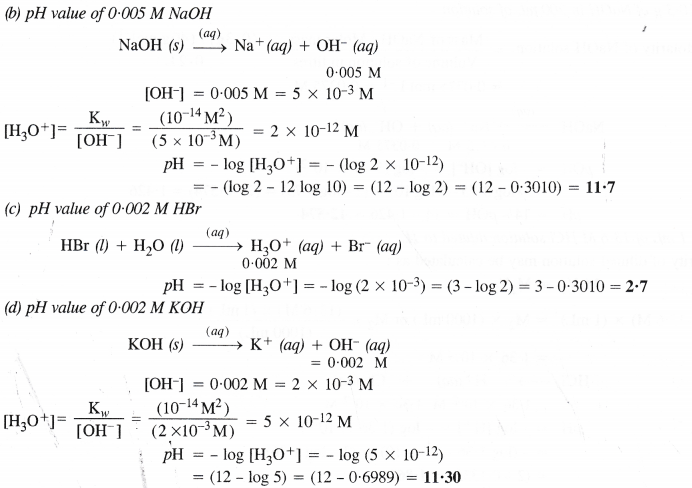Question 49.
Calculate the pH of the following solutions :
(a) 2 g of TlOH dissolved in water to give 2 litre of solution.
(b) 0.3 g of Ca(OH)2 dissolved in water to give 500 mL of solution.
(c) 0.3 g of NaOH dissolved in water to give 200 mL of solution.
(d) 1 mL of 13.6 M HCl diluted with water to give 1 litre of solution.Question 50.
The degree of ionization of 0.1 M bromoacetic acid solution is 0.132. Calculate the pH of the solution and pKa of bromoacetic acid.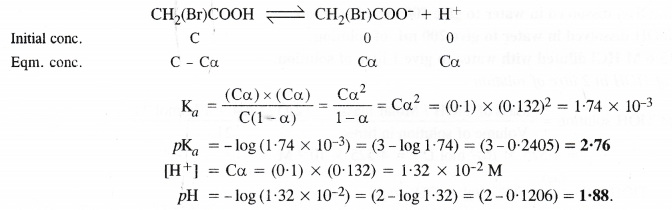Question 51.
The pH of 0-005 M codeine (C18H21NO3) solution is 9.95. Calculate its ionizationconstant and pKb.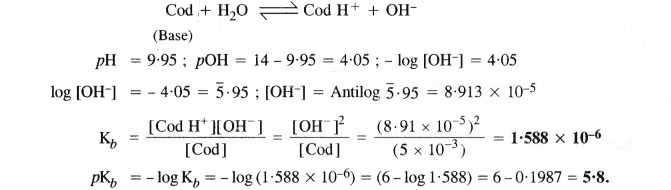Question 52.
What is the pH of 0.001 M aniline solution ? The ionization constant of aniline is 4.27 × 10-10. Calculate the degree of ionization of aniline in the solution. Also calculate the ionization constant of the conjugate acid of aniline.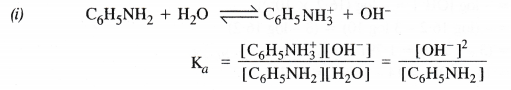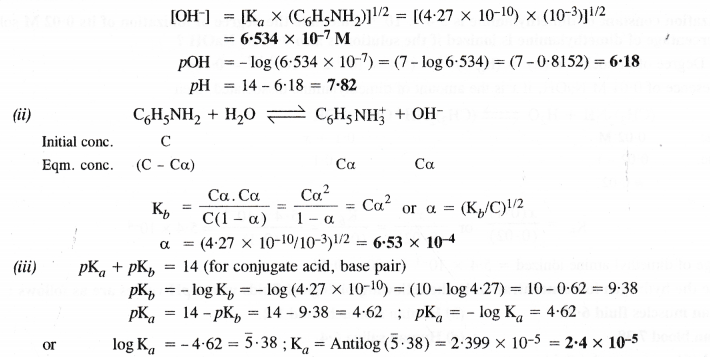Question 53.
Calculate the degree of ionization of 0.05 M acetic acid if its pKa value is 4.74. How is degree of dissociation affected when the solution contains (a) 0.01 M HCl (b) 0.1 M HCl ?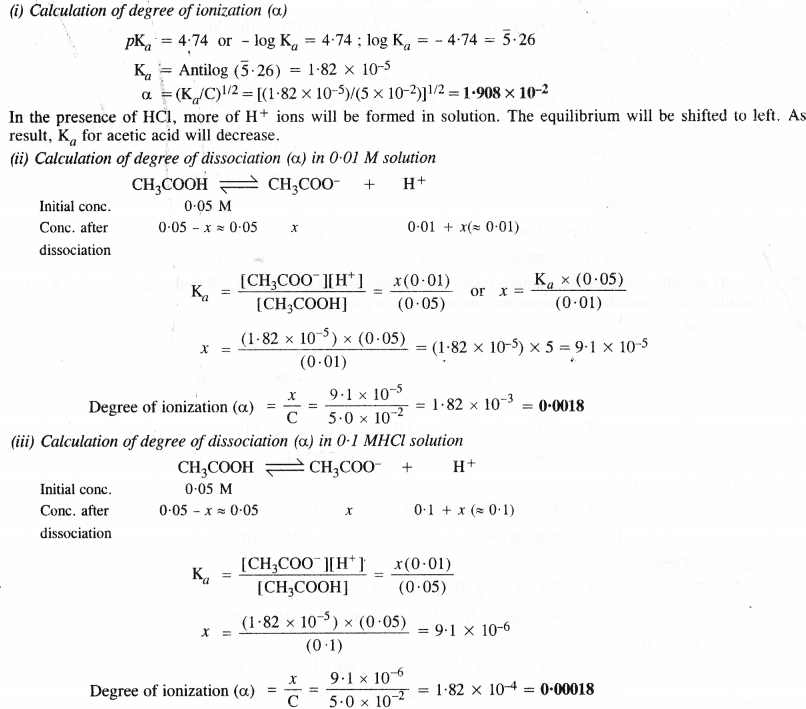Question 54.
The ionization constant of dimethylamine is 5.4 × 10-4. Calculate the degree of ionization of its 0.02 M solution. What percentage of dimethylamine is ionized if the solution is also 0.01 M NaOH ?Question 55.
Calculate the hydrogen ion concentration in the following biological fluids whose pH values are as follows :
(a) Human muscles fluid 6.83
(b) Human stomach fluid 1.2.
(b) Human blood 7.38
(d) Human saliva 6.4
(a) [H+] of human muscles fluid
pH = 6.83 or – log [H+] = 6.83 or log [H+] = – 6.83 = $$\bar { 7 }$$.17
[H+] = Antilog [H+] = Antilog [$$\bar { 7 }$$.17] = 1.479 ×10-7 M

(b) [H+ ] of human stomach fluid
pH = 1.2 or – log [H+] = 1.2 or log [H+] = – 1.2
[H+] = Antilog (-1-2) = Antilog (2-8) = 6.309 × 10-2 M

(c) [H+ ] of human blood
pH = 7.38 or – log [H+] = 7.38 or log [H+] = – 7.38
[H+] = Antilog (-7.38) = Antilog ($$\bar { 8 }$$.62) = 4.168 × 10-8 M

(d) [H+] of human saliva
pH = 6.4 or – log [H+] = 6.4 or log [H+] = – 6.4
[H+] = Antilog (-6.4) = Antilog ($$\bar { 7 }$$.6) = 3.98 × 10-7 M.

Question 56.
The pH of milk, black coffee, tomato juice, lemon juice and egg white are 6.8, 5.0, 4.2, 2.2 and 7.8 respectively. Calculate corresponding hydrogen ion concentration in each case.
(a) [H+] of milk
pH = 6.8 or – log [H+] = 6.8 or log [H+] = – 6.8
[H+] = Antilog (-6.80) = Antilog (7.20) = 1.58 × 10-7 M

(b) [H+] of black coffee
pH = 5.0 or – log [H+] = 5.0 or log [H+] = – 5.0
[H+] = Antilog (-5.0) = Antilog ($$\bar { 5 }$$) = 10-5 M

(c) [H+] of tomato juice
pH = 4.2 or – log [H+] = 4.2 or log [H+] = – 4.2
[H+] = Antilog (-4.2) = Antilog ($$\bar { 5 }$$.80) = 6.31 × 10-5 M

(d) [H+] of lemon juice
pH = 2.2 or – log [H+]= 2.2 or log [H+] = – 2.2
[H+] = Antilog (-2.2) = Antilog ($$\bar { 3 }$$.80) = 6.310 × 10-3 M

(e) [H+] of egg white
pH = 7.8 or – log [H+] = 7.8 or log [H+] = – 7.8
[H+] = Antilog (-7.8) = Antilog ($$\bar { 8 }$$.20) = 1.58 × 10-8 M

Question 57.
0.561 g KOH is dissolved in water to give 200 mL of solution at 298K. Calculate the concentration of potassium, hydrogen and hydroxyl ions. What is the pH of the solution ?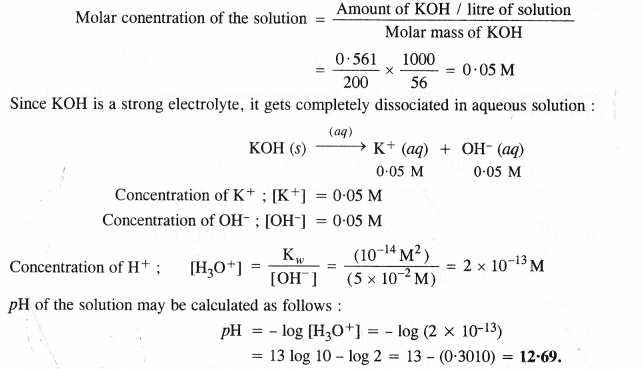Question 58.
The solubility of Sr(OH)2 at 298 K is 19.23 g/L of solution. Calculate the concentrations of strontium and hydroxyl ions and the pH of the solution. (Atomic mass of Sr = 87.6)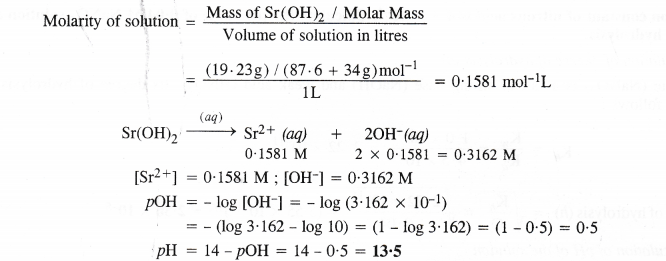Question 59.
The ionisation constant of propionic acid is 1.32 × 10-5. Calculate the degree of ionisation of acid in its 0.05 M solution and also its pH. What will be its degree of ionisation if the solution is 0.01 M in HCl also ?Question 60.
The pH of 0.1M solution of cyanic acid (HCNO) is 2.34. Calculate ionisation constant of the acid and also its degree of dissociation in the solution.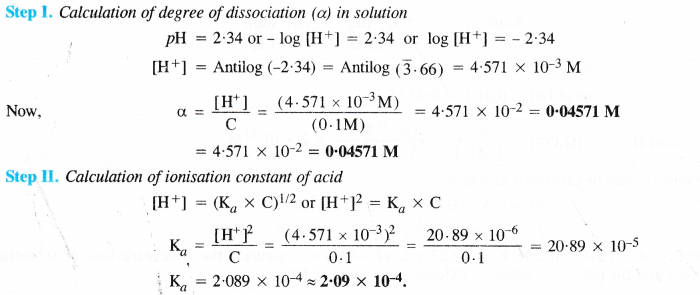Question 61.
The ionisation constant of nitrous acid is 4.5 × 10-4. Calculate the pH value of 0.04 M NaNO2 solution and also its degree of hydrolysis.Question 62.
A 0.02 M solution of pyridinium hydrochloride has pH = 3.44. Calculate the ionisation constant of pyridine.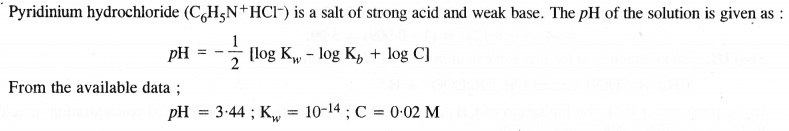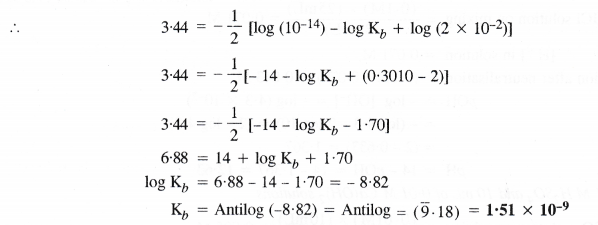Question 63.
Predict if the solutions of the following salts are neutral, acidic or basic :
NaCl, KBr, NaCN, NH4NO3, NaNO2, and KF
Neutral :NaCl, KBr
Basic : NaCN, NaNO2, KF
Acidic :NH4NO3

Question 64.
The ionisation constant of chloroacetic acid is 1.35 × 10-3. What will be the pH of 0.1 M acid solution and of its 0.1 M sodium salt solution ?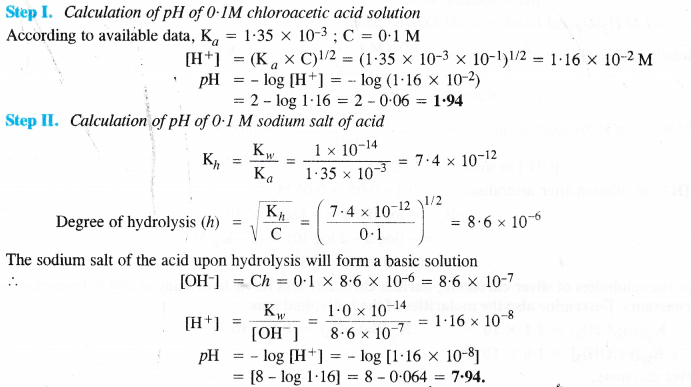Question 65.
The ionic product of water at 310 K is 2.7 × 10-14. What is the pH value of neutral water at this temperature ?
Since water is neutral ; [H3O+] = [OH]
We know that, [H3O+] × [OH] = Kw = 2.7 × 10-14
∴ [H3O+]2 = 2.7 × 10-14
or [H3O+] = (2.7 × 10-14)1/2 = 1.643 × 10-7
pH of water = – log [H3O+] = – log (1.643 × 10-7)
= (-) (-7) + log (1.643) = (7 – log 1.643) = 7 – 0.2156 = 6.78.

Question 66.
Calculate the pH of the resultant mixtures
(a) 10 mL. of 0.2 M Ca(OH)2 + 25 mL of 0.1 M HCl
(b) 10 mL of 0.01 M H2SO4 + 10 mL of 0.01 M Ca(OH)2
(c) 10 mL of 0.1 M H2SO4 + 10 mL of 0.1 M KOH.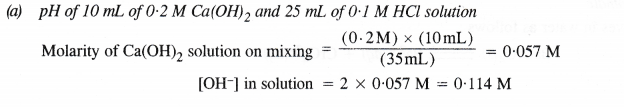Question 67.
Determine the solubilites of silver chromate, barium chromate and ferric hydroxide at 289 K from their solubility product constants. Determine also the molarities of the individual ions.
Given : KspAg2CrO4) = 1.1 × 10-12
Ksp(BaCrO4) = 1.2 × 10-10
Ksp[Fe(OH)3] = 1.0 × 10-38Question 68.
The solubility product constants of Ag2CrO4 and AgBr are 1.1 × 10-12 and 5.0 × 10-13 respectively. Calculate the ratio of the molarities of their saturated solutions.Question 69.
Equal volumes of 0.002 M solutions of sodium iodate and copper chlorate are mixed together. Will it lead to the precipitation of copper iodate ? (For copper iodate Ksp = 7.4 × 10-8).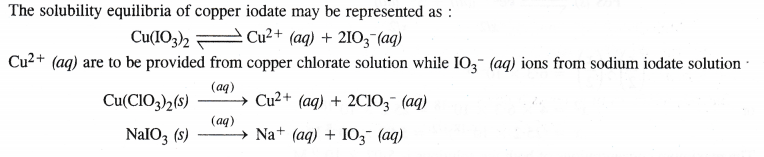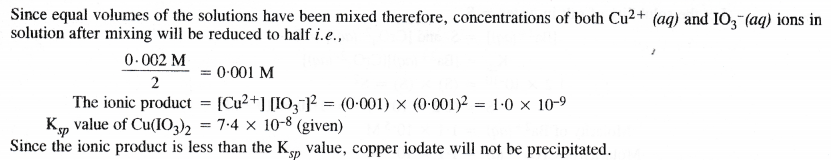Question 70.
The ionization constant of benzoic acid is 6.46 × 10-5 and Ksp for silver benzoate is 2.5 × 10-13. How many times is silver benzoate more soluble in a buffer of pH 3.19 compared to its solubility in pure water ?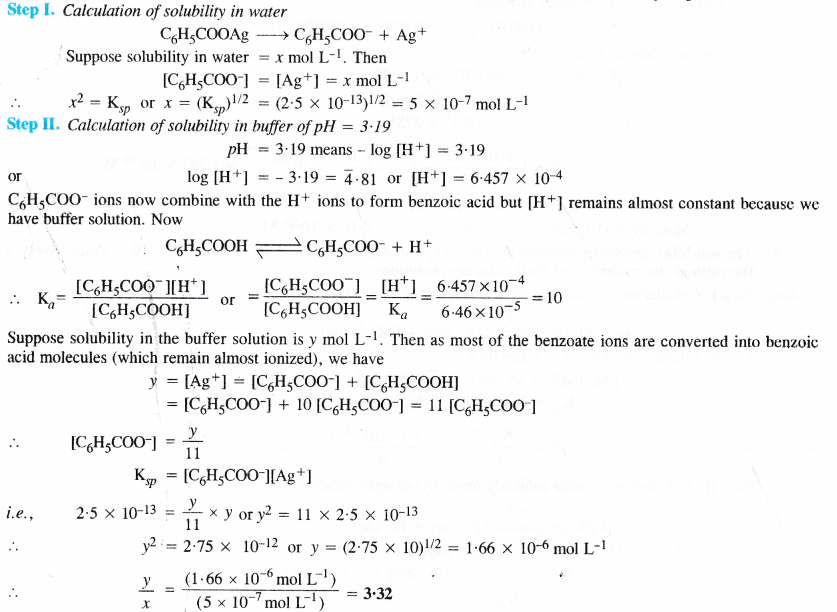Question 71.
What is the maximum concentration of equimolar solutions of ferrous sulphate and sodium sulphide so that when mixed in equal volumes, there is no precipitation of iron sulphide ? (For iron sulphide, Ksp = 6.3 × 10-18).